The MEANS Procedure

# Results: MEANS Procedure

PROC MEANS excludes missing values for the analysis variables before calculating statistics. Each analysis variable is treated individually; a missing value for an observation in one variable does not affect the calculations for other variables. The statements handle missing values as follows:

• If a class variable has a missing value for an observation, then PROC MEANS excludes that observation from the analysis unless you use the MISSING option in the PROC statement or CLASS statement.

• If a BY or ID variable value is missing, then PROC MEANS treats it like any other BY or ID variable value. The missing values form a separate BY group.

• If a FREQ variable value is missing or nonpositive, then PROC MEANS excludes the observation from the analysis.

• If a WEIGHT variable value is missing, then PROC MEANS excludes the observation from the analysis.

PROC MEANS tabulates the number of the missing values. Before the number of missing values are tabulated, PROC MEANS excludes observations with frequencies that are nonpositive when you use the FREQ statement and observations with weights that are missing or nonpositive (when you use the EXCLNPWGT option) when you use the WEIGHT statement. To report this information in the procedure output use the NMISS statistical keyword in the PROC statement.

You control the column width for the displayed statistics with the FW= option in the PROC statement. Unless you assign a format to a numeric class or an ID variable, PROC MEANS uses the value of the FW= option. When you assign a format to a numeric class or an ID variable, PROC MEANS determines the column width directly from the format. If you use the PRELOADFMT option in the CLASS statement, then PROC MEANS determines the column width for a class variable from the assigned format.

By default when you use a CLASS statement, PROC MEANS displays an additional statistic called N Obs. This statistic reports the total number of observations or the sum of the observations of the FREQ variable that PROC MEANS processes for each class level. PROC MEANS might omit observations from this total because of missing values in one or more class variables or because of the effect of the EXCLUSIVE option when you use it with the PRELOADFMT option or the CLASSDATA= option. Because of this action and the exclusion of observations when the WEIGHT variable contains missing values, there is not always a direct relationship between N Obs, N, and NMISS.

In the output data set, the value of N Obs is stored in the _FREQ_ variable. Use the NONOBS option in the PROC statement to suppress this information in the displayed output.

PROC MEANS can create one or more output data sets. The procedure does not print the output data set. Use PROC PRINT, PROC REPORT, or another SAS reporting tool to display the output data set.

Note:   By default the statistics in the output data set automatically inherit the analysis variable's format and label. However, statistics computed for N, NMISS, SUMWGT, USS, CSS, VAR, CV, T, PROBT, PRT,SKEWNESS, and KURTOSIS do not inherit the analysis variable's format because this format can be invalid for these statistics. Use the NOINHERIT option in the OUTPUT statement to prevent the other statistics from inheriting the format and label attributes.The output data set can contain these variables:

• the variables specified in the BY statement.

• the variables specified in the ID statement.

• the variables specified in the CLASS statement.

• the variable _TYPE_ that contains information about the class variables. By default _TYPE_ is a numeric variable. If you specify CHARTYPE in the PROC statement, then _TYPE_ is a character variable. When you use more than 32 class variables, _TYPE_ is automatically a character variable.

• the variable _FREQ_ that contains the number of observations that a given output level represents.

• the variables requested in the OUTPUT statement that contain the output statistics and extreme values.

• the variable _STAT_ that contains the names of the default statistics if you omit statistic keywords.

• the variable _LEVEL_ if you specify the LEVEL option.

• the variable _WAY_ if you specify the WAYS option.

The value of _TYPE_ indicates which combination of the class variables PROC MEANS uses to compute the statistics. The character value of _TYPE_ is a series of zeros and ones, where each value of one indicates an active class variable in the type. For example, with three class variables, PROC MEANS represents type 1 as 001, type 5 as 101, and so on.

Usually, the output data set contains one observation per level per type. However, if you omit statistical keywords in the OUTPUT statement, then the output data set contains five observations per level (six if you specify a WEIGHT variable). Therefore, the total number of observations in the output data set is equal to the sum of the levels for all the types you request multiplied by 1, 5, or 6, whichever is applicable.

If you omit the CLASS statement (_TYPE_= 0), then there is always exactly one level of output per BY group. If you use a CLASS statement, then the number of levels for each type that you request has an upper bound equal to the number of observations in the input data set. By default, PROC MEANS generates all possible types. In this case the total number of levels for each BY group has an upper bound equal to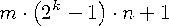where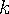is the number of class variables and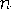is the number of observations for the given BY group in the input data set and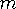is 1, 5, or 6.

PROC MEANS determines the actual number of levels for a given type from the number of unique combinations of each active class variable. A single level consists of all input observations whose formatted class values match.

The following figure shows the values of _TYPE_ and the number of observations in the data set when you specify one, two, and three class variables.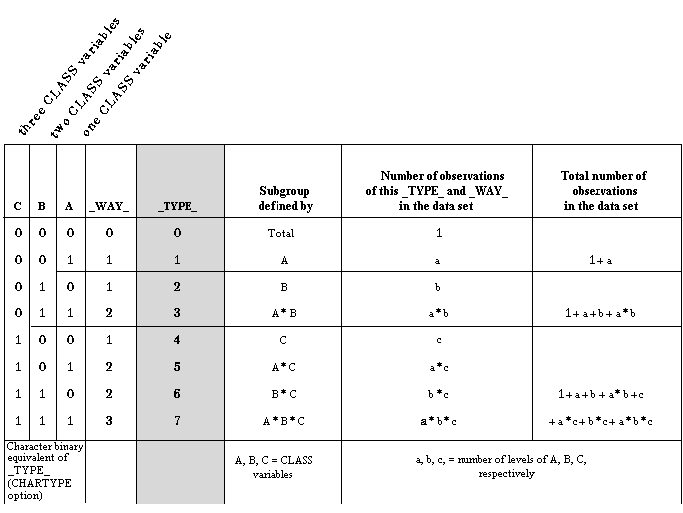Previous Page | Next Page | Top of Page